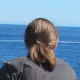Visualizing the R packages galaxy

# Visualizing the R packages galaxy

The idea of this post is to create a kind of map of the R ecosystem showing dependencies relationships between packages. This is actually quite simple to quickly generate a graph object like that, since all the data we need can be obtained with one call to the function `available.packages()`. There are a few additional steps to clean the data and arrange them, but with the following code everyone can generate a graph of dependencies in less than 5s.

First, we load the igraph package and we retrieve the data from our current repository.

```library(igraph)
dat <- available.packages()
```

For each package, we produce a character string with all its dependencies (Imports and Depends fields) separated with commas.

```dat.imports <- paste(dat[, "Imports"], dat[, "Depends"], sep = ", ")
dat.imports <- as.list(dat.imports)
dat.imports <- lapply(dat.imports, function(x) gsub("\\(([^\\)]+)\\)", "", x))
dat.imports <- lapply(dat.imports, function(x) gsub("\n", "", x))
dat.imports <- lapply(dat.imports, function(x) gsub(" ", "", x))
```

Next step, we split the strings and we use the stack function to get the complete list of edges of the graph.

```dat.imports <- sapply(dat.imports, function(x) strsplit(x, split = ","))
dat.imports <- lapply(dat.imports, function(x) x[!x %in% c("NA", "R")])
names(dat.imports) <- rownames(dat)
dat.imports <- stack(dat.imports)
dat.imports <- as.matrix(dat.imports)
dat.imports <- dat.imports[-which(dat.imports[, 1]==""), ]
```

Finally we create the graph with the list of edges. Here, I select the largest connected component because there are many isolated vertices which will make the graph harder to represent.

```g <- graph.edgelist(dat.imports)
g <- decompose.graph(g)
g <- g[[which(sapply(g, vcount) == max(sapply(g, vcount)))]]
```

Now that we have the graph we can compute many graph-theory related statistics but here I just want to visualize these data. Plotting a large graph like that with `igraph` is possible but slow and tedious. I prefer to export the graph and open it in Gephi, a free and open-source software for network visualization.
The figure below was created with Gephi. Nodes are sized according to their number of incoming edges. This is pretty useless and uninformative, but still I like it. This looks like a sky map and it is great to feel that we, users and developers are part of this huge R galaxy.

## 5 réactions au sujet de « Visualizing the R packages galaxy »

1.Joshua dit :

Nice post! And nice name too!

2.Ilya dit :

Would be cool to make it interactive — put a mouse pointer over a node and package name appears (maybe also some basic info, like how old the package is, who is the author, etc.)

1.franzk dit :

Sure. I didn’t try to make it interactive but it would be easy to link to basic info you can get from available.packages().

3.Anubhav dit :

Hi,

I was really impressed by the visualization, but I am unable to do so using Gephi, could you tell me how I can re create the plot you did.

Thanks a lot for sharing this!

1.franzk dit :

Hi, it’s not really easy to reproduce results in Gephi.
As I remember, I used the Force Atlas 2 layout. Nodes size and color depend on incident edges numbers (this is a white-blue gradient with a spline transformation). Finally note that I added blur effect on the nodes with Gimp. I hope that helps.